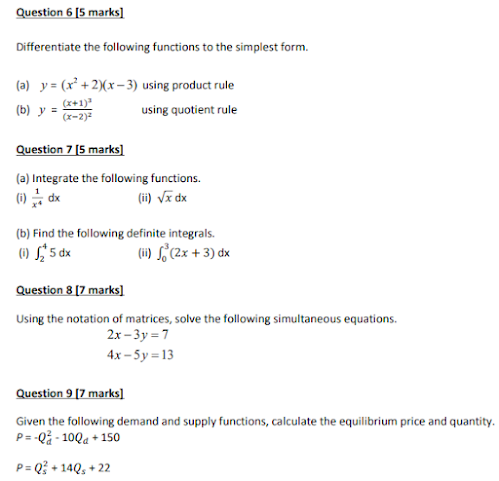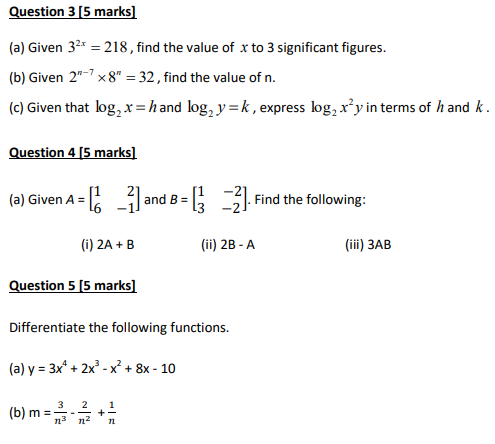Aug 03, 2023

Question 1

Evaluate without a calculator, using the BODMAS order of operations

Question 2

It is given that three points, P(4,6), Q(2,-2) and R(-5, 7) lie on a Cartesian plane. S is the midpoint of the straight line PQ. Find

(a) the gradient of the straight line SR.

(b) the equation of the straight line SR.Question 10

Given the price function, P = 100 – Q and the total cost function, TC = 2Q
2 + 10Q + 13. Find

(a) total revenue, TR function

(b) total profit, π function

Question 11

A bag contains 10 balls. 6 of the balls are blue balls while the remaining are red balls. Two balls are taken at random from the bag, one after another without replacement. Calculate the probability that

(a) both balls are blue balls.

(b) both balls taken are of different colour.

Question 12

John started working for a company on 1 January 2013 with an annual salary of RM24000. Every January, the company increased her salary by 6% of the previous year’s salary. Calculate

(a) his annual salary, to the nearest RM, for the year 2020.

(b) the total salary, to the nearest RM, paid to him by the company, for the years 2013 to 2020.

Question 13

The quantity supplied for a product is 100 units at a price of \$20. However, when the price rises to \$30, the quantity supplied increases to 200 units.

(a) Express the linear demand function, Q in terms of quantity demanded, P.

(b) Determine the price that would result in a quantity supplied of 300 units.

(c) Interpret the slope and y-intercept.

Question 14

The manager of Hudson Auto would like to gain a better understanding of the cost of parts used in the engine tune-ups performed in the shop. She examines 30 customer invoices for tune-ups. The costs of parts, rounded to the nearest RM, are listed below.

155 131 135 150 133 145
129 146 116 118 116 137
127 108 134 159 123 147
143 100 130 155 153 138
141 158 136 126 144 130

(a) Construct a frequency distribution table.

(b) Using Excel, construct a histogram.

(c) Find all the three measures of central tendency.

(d) Find the first and third quartile.

Question 15

The following table shows the size of companies and whether they offer stock options to their board members as part of their non-cash compensation packages.

A random company is selected. Find the probability that the company

(a) offers stock options to their members.
(b) is large and offer stock options to their members.
(c) is small or offer stock options to their members.
(d) is medium given that it offers stock options to their members.